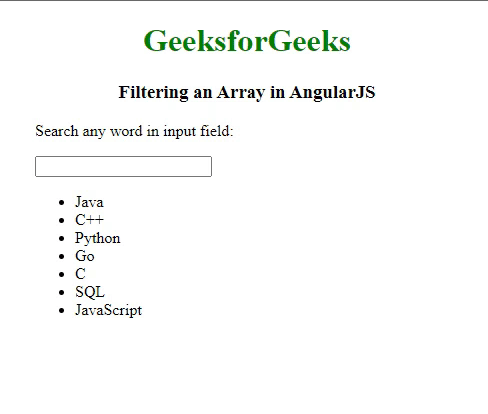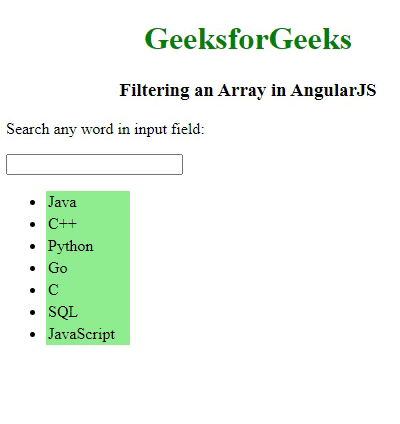Open in App
Not now

# How to Filter an Array based on user input in AngularJS ?

• Difficulty Level : Hard
• Last Updated : 14 Nov, 2022

AngularJS is a JavaScript-based framework. It can be used by adding it to an HTML page using a <script> tag. AngularJS helps in extending the HTML attributes with the help of directives and binding of data to the HTML with expressions. Angular filters can be added in AngularJS to format data.

In this article, we will be discussing how to filter an array based on user input in AngularJS. Filtering in angular works by typing a letter in the input field and the list will shrink or grow based on the matched results.

Filters in Angular: AngularJS provides users to use filters to transform data like ‘currency’ which formats a number to a currency format, ‘date’ which formats a date to a specified format, etc. Filters in angular can be added to an expression or directives using the pipe | symbol as shown below.

`{{ expression | filterName:parameter }}`

To filter an array of data based on the user’s input, we use the ‘ng-model’ directive by setting it on an input field. After which, we can use the value of the input field as an expression in the filter. So, to filter an array, all we need is an array of data and an input field to collect the user input.

The ng-model directive binds the values of the HTML controls like input, select, etc, and stores the required user value in a variable that can be used whenever we require that value. It is mostly supported by <input>, <select>, and <textarea>. For creating the filter to search for an array of data, we’ll be using this directive of angular.

Syntax:

```<div ng-app="myApp" ng-controller="namesCtrl">
<p>
<input type="text" ng-model="test">
</p>
<ul>
<li ng-repeat="letter in names | filter : test">
{{ letter }}
</li>
</ul>
</div>```

Example 1: The below example demonstrates how to filter an array based on the user input in HTML. When we click in the Input field and try typing any letter in the input field, we will see that the list will go shrinking if the match results of the typed letter get matched.

## HTML

 ```<``html` `lang``=``"en"``>`` ` `<``head``>``    ``<``meta` `charset``=``"utf-8"` `/>``    ``<``title``>GeeksforGeeks``    ``<``script` `src``=``"https://ajax.googleapis.com/ajax/libs/angularjs/1.6.9/angular.min.js"``>``    `````` ` `<``body``>``    ``<``div` `style``=``"text-align: center"``>``        ``<``h1` `style``=``"color: green"``>GeeksforGeeks``        ``<``h3``>Filtering an Array in AngularJS``    ````    ``<``div``>``        ``<``div` `ng-app``=``"gfgApp"` `             ``ng-controller``=``"langsCtrl"``>``            ``<``p``>Search any word in input field:``            ``<``p``>``                  ``<``input` `type``=``"text"` `ng-model``=``"test"` `/>``              ````            ``<``ul``>``                ``<``li` `ng-repeat``=``                ``"word in lang | filter:test"``>``                    ``{{ word }}``                ````            ````        ```` ` `        ``<``script``>``            ``angular.module("gfgApp", []).controller("langsCtrl", ``            ``function (\$scope) {``              ``\$scope.lang = ["Java","C++","Python",``                             ``"Go","C","SQL","JavaScript",``              ``];``            ``});``        ````    `````` ` ``

Output:Example 2: The below example demonstrates a customized array-based filtration on the user input in HTML, where the list of arrays is customized and filtered based on the user input.

## HTML

 ```<``html` `lang``=``"en"``>`` ` `<``head``>``    ``<``meta` `charset``=``"utf-8"` `/>``    ``<``title``>GeeksforGeeks``    ``<``script` `src``=``"https://ajax.googleapis.com/ajax/libs/angularjs/1.6.9/angular.min.js"``>`` `````` ` `<``body``>``    ``<``div` `style``=``"text-align: center"``>``        ``<``h1` `style``=``"color: green"``>GeeksforGeeks``        ``<``h3``>Filtering an Array in AngularJS``    ````    ``<``div``>``        ``<``div` `ng-app``=``"gfgApp"` `ng-controller``=``"langsCtrl"``>``            ``<``p``>Search any word in input field:``            ``<``p``>``                ``<``input` `type``=``"text"` `ng-model``=``"test"` `/>``            ````            ``<``ul``>``                ``<``li` `ng-repeat``=``"word in lang | filter:test"` `                    ``style="background-color: lightgreen; ``                           ``width: 5rem; padding: 2px">``                    ``{{ word }}``                ````            ````        ```` ` `        ``<``script``>``            ``angular.module("gfgApp", []).controller("langsCtrl", ``            ``function (\$scope) {``              ``\$scope.lang = ["Java","C++","Python","Go",``                             ``"C","SQL","JavaScript"];``            ``});``        ````    `````` ` ``

Output:My Personal Notes arrow_drop_up